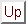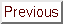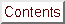Next: About the CONDOR algorithm. Up: An introduction to the Previous: A simple trust-region algorithm.   Contents

# The basic trust-region algorithm (BTR).

Defnition: The trust region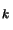is the set of all points such that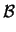(2.18)

The simple algorithm described in the Section 2.2 can be generalized as follows:
1. Initialization An initial pointand an initial trust region radiusare given. The constants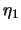,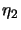,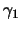and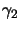are also given and satisfy: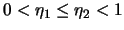and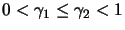(2.19)

Compute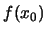and set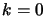2. Model definition Choose the norm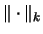and define a model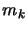in3. Step computation Compute a step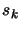that ''sufficiently reduces the model''and such that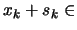4. Acceptance of the trial point. Compute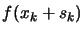and define:(2.20)

If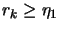, then define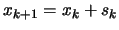; otherwise define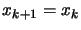.
5. Trust region radius update. Set(2.21)

Increment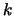by 1 and go to step 2.
Under some very weak assumptions, it can be proven that this algorithm is globally convergent to a local optimum [CGT00a]. The proof will be skipped.Next: About the CONDOR algorithm. Up: An introduction to the Previous: A simple trust-region algorithm.   Contents
Frank Vanden Berghen 2004-04-19